Select Page

Common Core Standard: 8.F.A.2, 8.F.A.3

Use: Individually as a quick Sticky Math Warmup, in groups for a longer Sticky Math Warmup, or all together as a Sticky Math Lesson

Note: Post It sells sticky notes with grids on them, which makes using Sticky Math with graphing much simpler.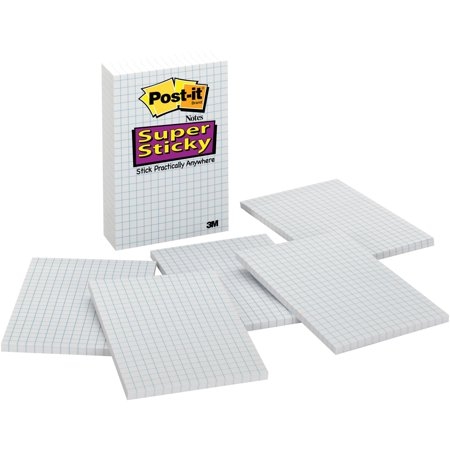These Sticky Math activities can stand alone; however, it is a continuation of the post Slope Intercept Form; hence, it begins with stage four.

STAGE FOUR: EXPLORING THE DIFFERENT PLACEMENTS OF THE NEGATIVE SIGN

This set consists of a Sticky Math Triad, a Sticky Math Pair and a Sticky Math Comparison.  The point of all three is to realize that wherever a single negative sign is placed the result is a negative slope.  The goal is not to memorize this as a fact; students should be able to explain why.  Students / teachers focused only on “rise over run” may miss this in the Sticky Math Triad.  The Sticky Math Pair that follows might lead to this realization as the negative sign is in neither the numerator nor the denominator.  If this fact has not been discussed by the Sticky Math Comparison, then teachers should pose purposeful questions to draw it out.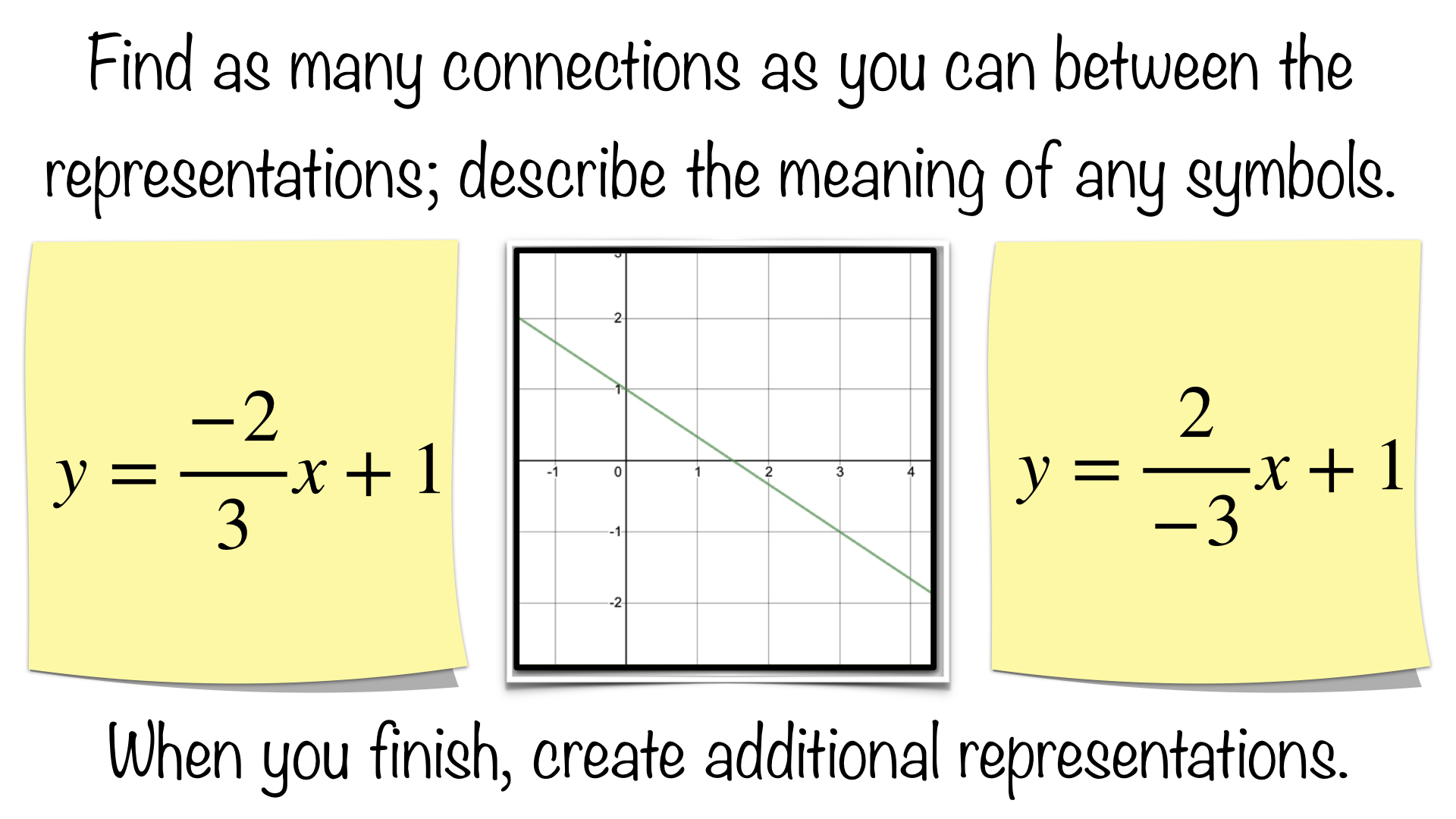Connections could include:

• Any connections from previous stages
• The graph is a representation of the left sticky
• The graph is a representation of the right sticky
• Employing “rise over run” on the left sticky moves you left to right down the line
• Employing “rise over run” on the right sticky moves you right to left up the line
• The graph is a representation of BOTH stickies

Students could create their own representations; these might include:

• any of the representations from previous stages
• putting the negative sign in front of the slope fraction like the Sticky Math Pair that follows
• a graph with a different scale
• a t-chart or table of values
• for the left sticky, “I started with one dollar.  I owe 2 dollars (-2) every 3 days.”
• for the right sticky, “I started with one dollar.  I had 2 dollars more 3 days ago (-3).

Students may not come up with the final two bullets.  You could introduce them one at a time on new stickies asking which representation they describe.  While they fit one equation literally, each one fits all the representations.  If you owed 2 dollars more every 3 days, then you would have had 2 dollars more 3 days ago.  How is the next Sticky Math Pair the same too?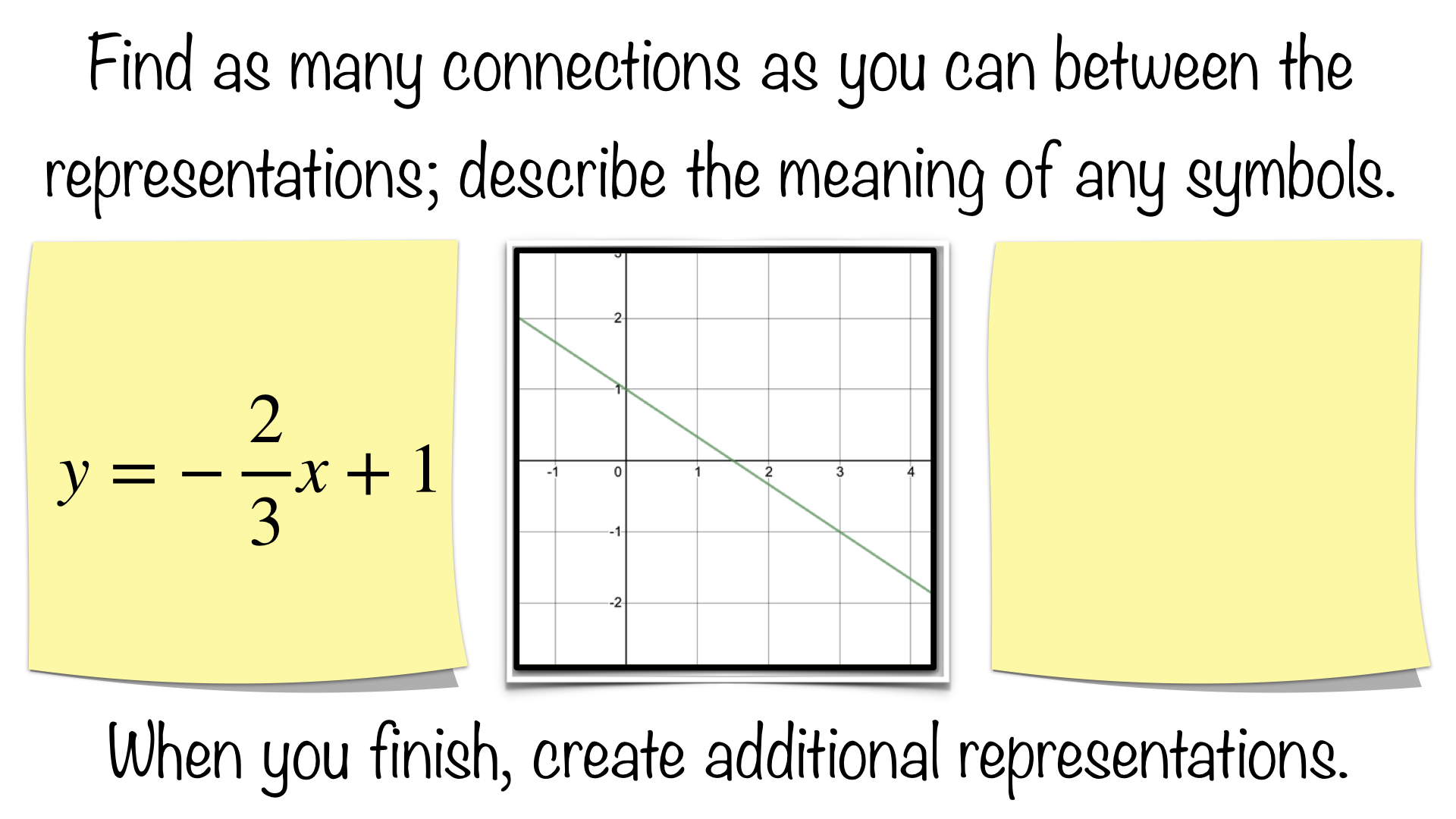Connections could include:

• any connections from previous stages
• the graph is a representation of the left sticky
• If you think of the negative being in the numerator, then the it moves you left to right down the line
• If you think of the negative being in the denominator, then the it moves you right to left up the line
• the negative is on the coefficient of x; thus, it is a multiplier of x.  As x increases, the entire term becomes more negative, which decreases the value of the expression and y.

The final bullet above is a key understanding conceptually to build fluency.  If it is not made here, then it needs to be made on the Sticky Math Comparison that follows.

Students could create their own representations; these might include:

• any of the representations from previous stages
• a new equation with the negative in the numerator
• a new equation with the negative in the denominator
• a graph with a different scale
• a t-chart or table of values
• “I started with one dollar.  I got in debt 2/3 of a dollar each day.”

Students may not come up with the last bullet above. Relating it to the discussion immediately preceding this stage: if you owed 2 dollars more every 3 days, then you would have had 2 dollars more 3 days ago. If I owe 2 dollars more every three days, then how much do I owe EACH day?  Posing this purposeful question can create an opportunity to explore and build fractional understanding.  The question could be rephrased, “what is amount is repeated 3 times to yield 2 dollars?”  As an equation, (x)3 = 2 where x is 2/3 so (2/3)3 = 2, which brings in a discussion of the multiplying of fractions. Or, thinking of it as addition, 2/3 + 2/3 + 2/3 = 6/3 or 2.

You could also introduce a sticky like the one on the left below, and discuss its meaning by posing purposeful questions: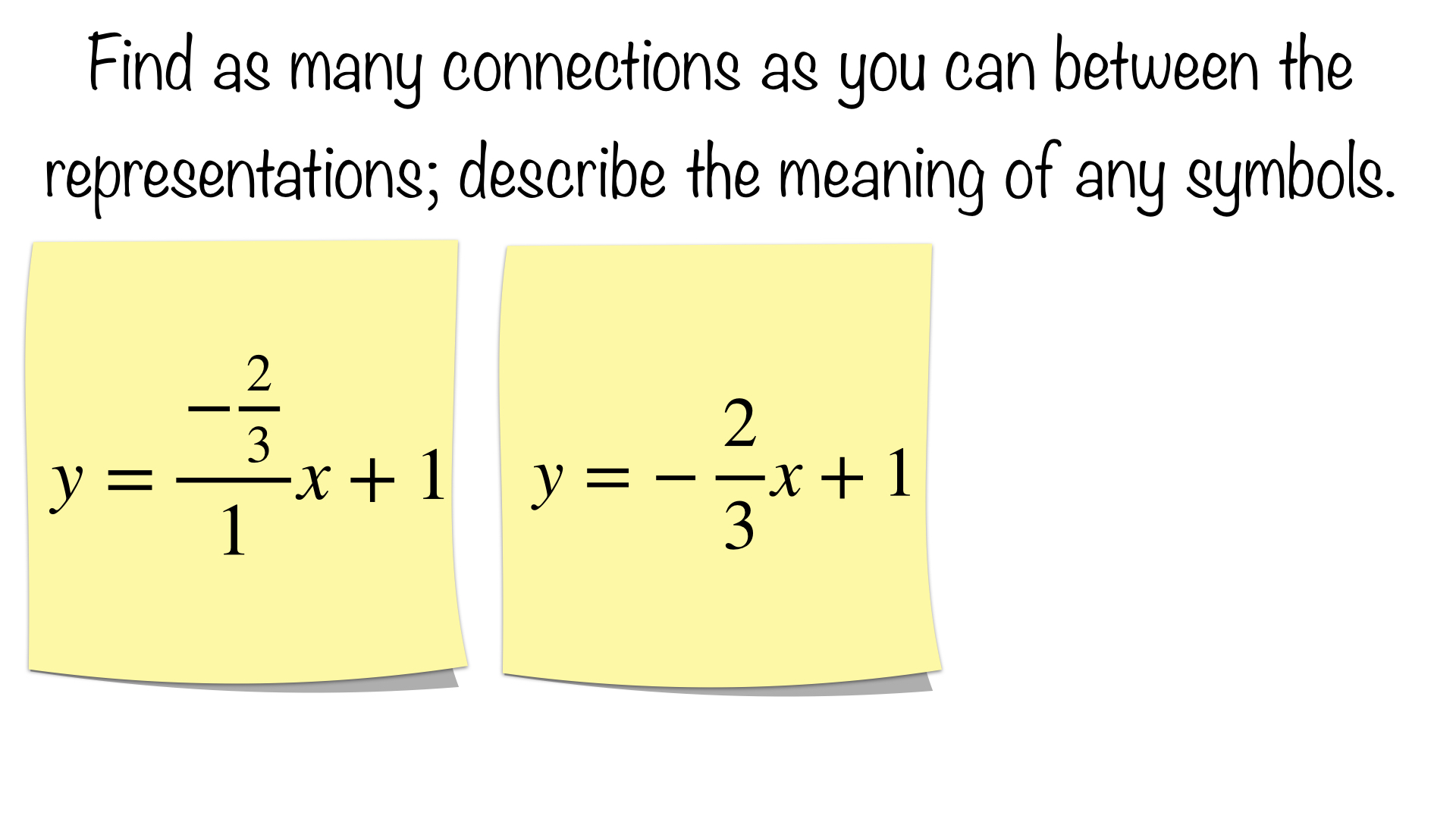Notice by creating the complex fraction the negative has been assigned to the numerator, which aids in seeing it as a single quantity for those who have only learned “rise over run” as a procedure.  It also make the description, “I started with one dollar.  I owe 2/3 of a dollar each (1) day.” more explicit by including the one day.  Incidentally, this a chance to reengage with complex fractions without going back to reteach seventh grade.  Remind students that it is always one quantity no matter where the negative sign is written, which is the point of the Sticky Math Comparison that follows.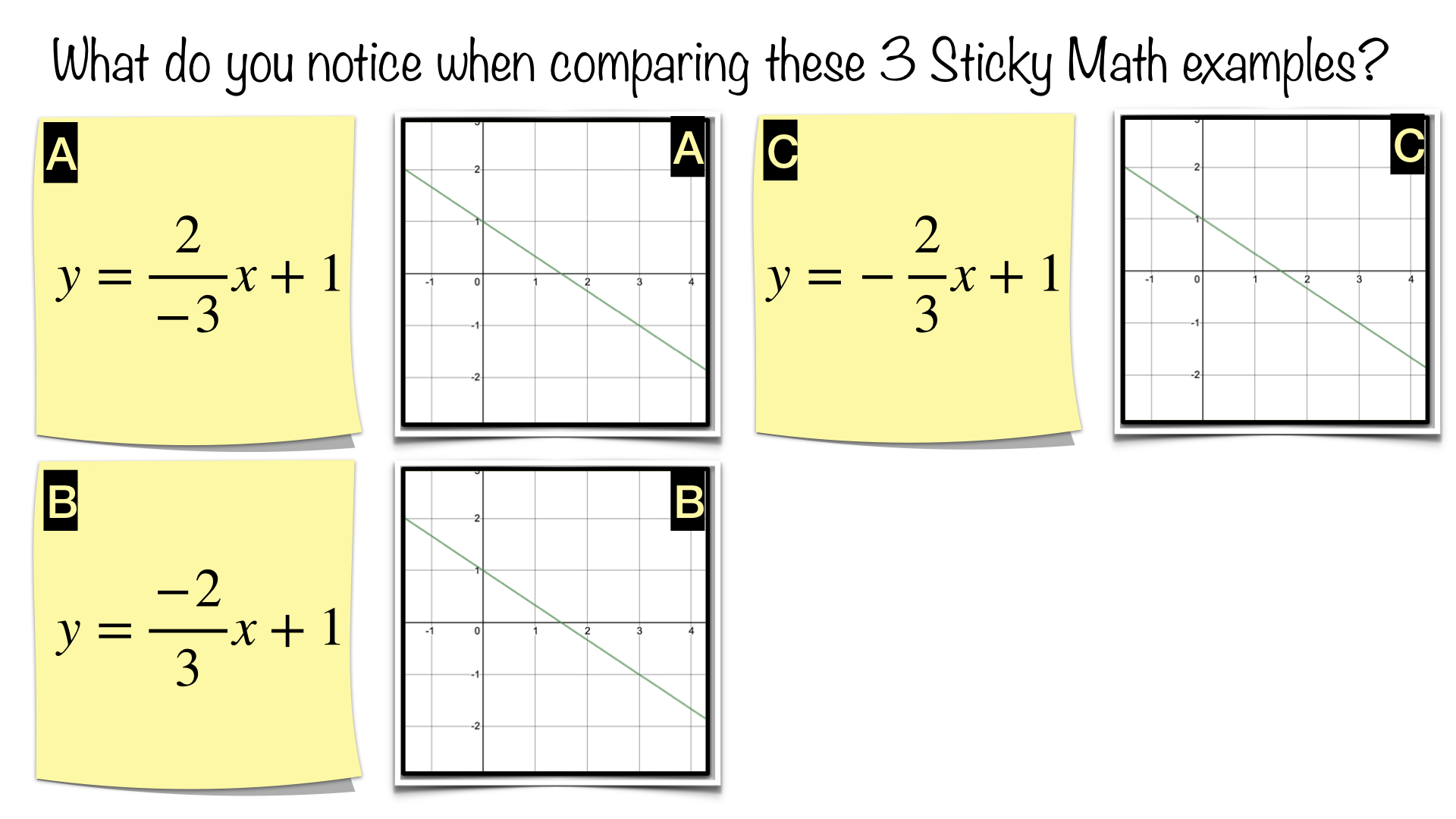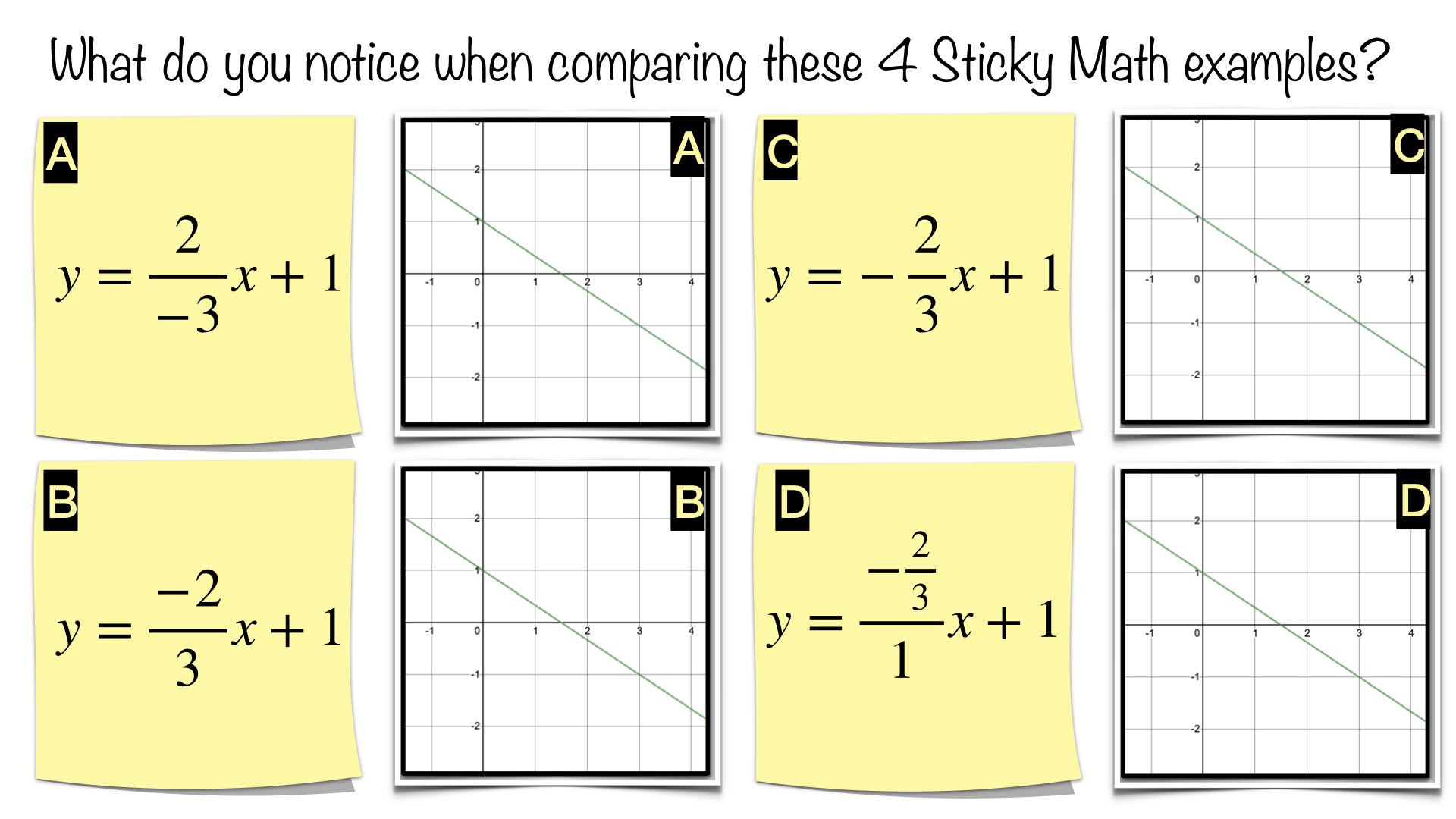These Sticky Math Comparisons are one last chance to make the connections discussed previously. Choose one depending on the discussion you have had to this point or want to have at this point. Either can be used by the teacher as a formative assessment as to whether or not students have internalized the connections discussed previously.  The main point is that no matter where the negative is placed on the coefficient of x, it is a multiplier of x.  As x increases, the entire term becomes more negative, which decreases the value of the expression and y.

STAGE FIVE: WHAT WOULD TWO NEGATIVE SIGNS MEAN ON THE SLOPE FRACTION?

Why should we even care what the right sticky means below?  Can’t we just say, “the opposite of a negative is a positive” and move on?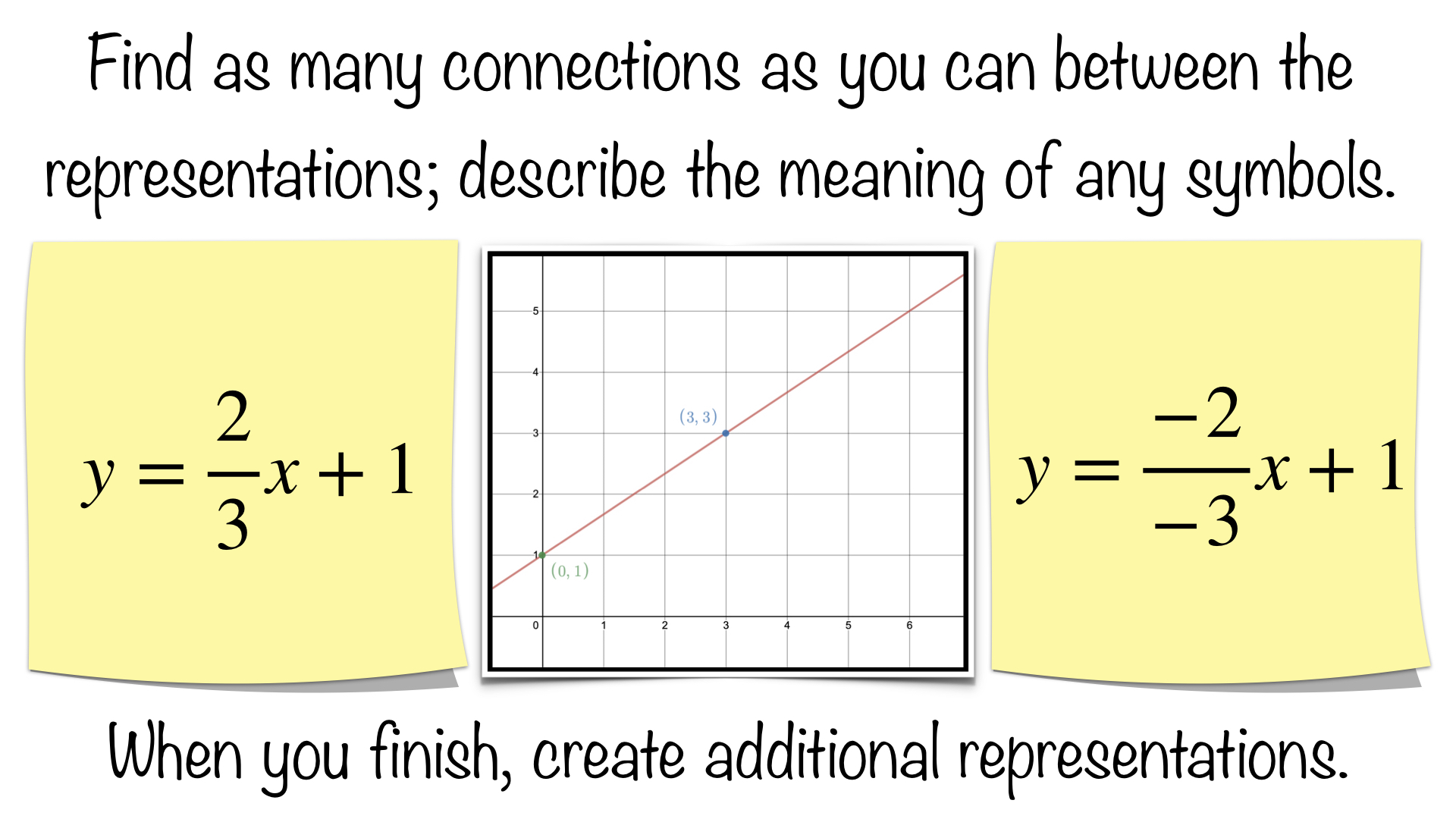Being able to convert a positive slope into a double negative can help students graph points using only slope intercept form moving in the “opposite” direction.  For an example, see the first two connections below:

Connections could include:

• the left sticky, takes you up the line from left to right
• the right sticky, takes you down the line from right to left
• the graph is a representation of the both stickies
• any other connection from previous stages
• both right and left stickies have a y-intercept of 1
• all three stickies represent the same function

Students could create their own representations; these might include:

• any of the representations from previous stages
• a t-chart or a table of values
• for the left sticky, “I had 1 dollar.  I received 2 dollars every 3 days.”
• for the right sticky, “I had 1 dollar.  3 days ago (-3), I had 2 dollars less (-2).”

Like previous posts, students may not come up with the last two bullets; however, you can introduce them one at a time if you like on individual stickies.  While literally one verbal description fits the left sticky and the other fits the right, they are actually describing the same event differently; they are equivalent expressions.  If you received 2 dollars every 3 days, then 3 days ago (-3) you had 2 dollars less (-2).

STAGE SIX: PUTTING IT ALL TOGETHER

The Sticky Math Comparison below allows students to share what they have learned in previous stages acting as a formative assessment for teachers while demonstrating intentionally that the presence of a negative in the numerator and the denominator is not equivalent to the presence of a single negative.Connections could include:

• any connection from previous stages
• Examples A, B & C are all have one negative sign
• Example D has two negative signs
• Examples A, B & C are all equivalent
• Example D shows an opposite relationship from the rest, gaining 2 dollars every 3 days rather than losing it (see the final paragraph of stage 5 for more on this)

A closing, formative assessment activity might be to complete a Sticky Math Matching activity like the one below:Any stickies from the six stages could be used to create a Sticky Math Matching activity.  Four representations for each function have been used here.  You could build up to this by including t-charts, table of values, or verbal descriptions in the discussions to this point as mentioned above.

Some purposeful questions you might pose about the t-charts / table of values

• “What is the benefit of choosing the values for x that they choose in the top t-chart?”
• “Where is the slope of 2/3 on the top t-chart?”
• “Where is the intercept on the top t-chart?”
• “Where is the slope found on the bottom t-chart?”
• “Where is the intercept on the bottom t-chart?”
• Could both t-charts be combined to create a single t-chart?  Why or why not?  Explain.
• Are the slopes the same in both t-charts?  Why or why not?  Explain.

The addition of the t-charts / table of values leads to the exploration of slope as the change in y over the change in x.  Rate of change should be emphasized by seeing it as how you move from one point to the other.  The fact that a t-chart / table of values like these are made up of individual points should also be stressed.  Another realization is that, in this case, the entire t-chart . table of values represents a line or linear function.

Lesson extension, classwork or homework – give each students 8 stickies have them create their own matching game to illustrate two different linear relationships; or, if you have done the work, then one linear relationship and one non-linear relationship.

Have them give it to a family member to complete, and then they check it.  Have students exchange them when they are finished or return to class, match the pairs, and check each other’s work as a ticket out the door or an opening activity the following day.

One final word on slope and graphing, I hope you have seen through this blog post that being procedurally proficient with “rise over run” is not sufficient by itself to achieve procedural fluency.  Besides being sometimes misleading, negative slopes are not rising, it does not account for a negative that is neither in the numerator nor the denominator.  Different representations beg for different ways to view slope;

• in an equation like y=mx+b, m might refer to how one moves between points where x and y together represent all the points (x,y) and b might represent a place to begin when graphing utilizing the y-intercept
• in a graph rise over run might be appropriate if one understands that a negative rise is possible and that one might run forward or backwards depending on the sign
• in a t-chart or table of values, change in y over the change in x is helpful
• in a verbal description, a rate of change is helpful
• all of the above are rates of change and are equivalent

exploring each of these conceptually should improve procedural fluency.

Common Core Standards: 8.F.A.2, 8.F.A.3

What connections are your students making?  What modifications are you making to use this with students?  We would love to hear your feedback; please submit a comment below or consider submitting your own Sticky Math activity here.